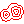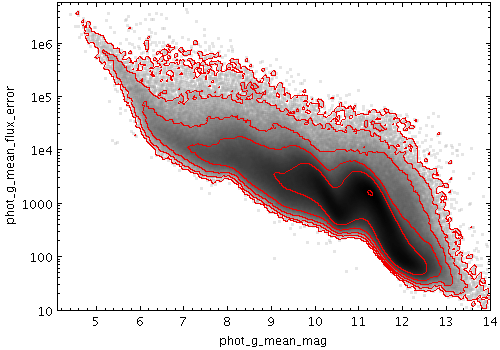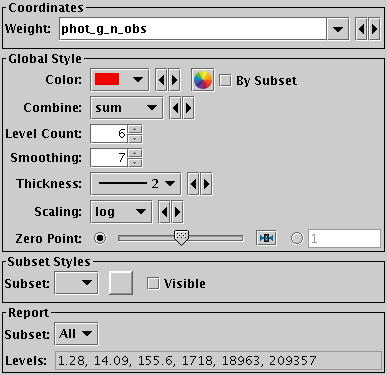Next Previous Up Contents
Next: Grid Form
Up: Plot Forms
Previous: Text Label Form

#### A.4.5.17 Contour Form

The Contour form () plots position density contours. These provide another way (alongside the Auto, Density and Weighted shading modes) to visualise the characteristics of overdense regions in a crowded plot.

The contours are currently drawn as pixels rather than lines, so they don't look very beautiful in exported vector output formats (PDF, PostScript).Example Contour plotContour form configuration panel

The configuration options are:

Weight
The weighting to apply to each input sample when producing the grid whose values are to be contoured. If supplied this is used in conjunction with the Combine selection. If left blank, an effective weighting of unity is used, and only smoothed point densities are contoured.
Color
The colour of the contour lines. If overlaid on top of other plot types that use the same colour, you may want to change this from its default value.
Combine
Defines the way that the weight values are combined when generating the value grid for which the contours will be plotted. If a weighting is supplied, the most useful values are `mean` which traces the mean values of a quantity and `sum` which traces the weighted sum. Other values such as `median` are of dubious validity because of the way that the smoothing is done.

This value is ignored if the weighting coordinate Weight is not set.

The available options are:

• `sum`: the sum of all the combined values per bin
• `mean`: the mean of the combined values
• `median`: the median of the combined values (may be slow)
• `stdev`: the sample standard deviation of the combined values
• `min`: the minimum of all the combined values
• `max`: the maximum of all the combined values
• `count`: the number of non-blank values per bin (weight is ignored)

Level Count
The number of contours drawn. In fact this is an upper limit; if there is not enough variation in the plot's density, then fewer contour lines will be drawn.
Smoothing
The size of the smoothing kernel applied to the density before performing the contour determination. If set too low the contours will be too crinkly, and if too high they will lose definition.
Scaling
How the smoothed density is treated before the contours levels are determined. Options are linear, logarithmic and equal area.
Zero Point
Determines the level at which the first contour (and hence all the others, which are separated from it by a fixed amount) are drawn. By sliding this from side to side you can sweep the contours over the density range and get a good idea of where interesting features lie.
The plot reports the contour levels it has used:
Levels
The actual values at which contours have been plotted. This can be useful for weighted plots using with a Combine value of mean, but for other combinations it may not have much physical meaning.

Next Previous Up Contents
Next: Grid Form
Up: Plot Forms
Previous: Text Label Form

TOPCAT - Tool for OPerations on Catalogues And Tables